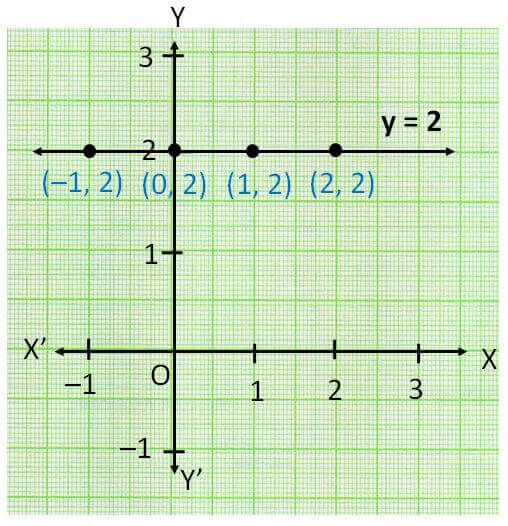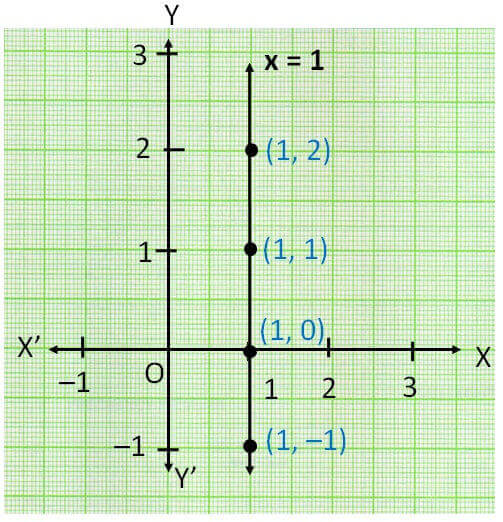Lines parallel x or y axis

Chapter 4 Class 9 Linear Equations in Two Variables
Concept wise

## Lines parallel to x-axis

Equation of x-axis is

y = 0

Equation of lines which are parallel to x-axis is

y = k

where k is a constant

Let us check an example

y = 2

Drawing y = 2 in graph

Since y = 2 for all values of x

Points which satisfy the equation is

 x 0 1 2 –1 y 2 2 2 2

Plotting points on graphHence, y = 2 is  line which is parallel to x axis

## Lines parallel to y-axis

Equation of y-axis is

x = 0

Equation of lines which are parallel to y-axis is

x = k

where k is a constant

Let us check an example

x = 1

Drawing x = 1 in graph

Since x = 1 for all values of y

Points which satisfy the equation is

 x 1 1 1 1 y 0 1 2 –1

Plotting points on graphHence, x = 1 is  line which is parallel to x axis

Get live Maths 1-on-1 Classs - Class 6 to 12